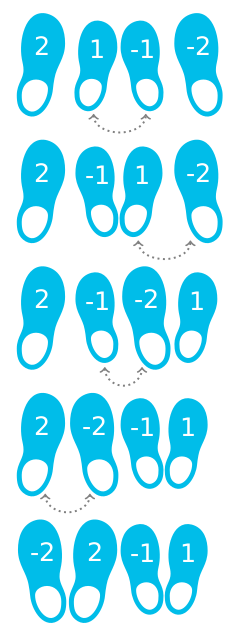# #533. 【IOI2019】排列鞋子

Adnan 拥有巴库最大的鞋店。现在有一个装着 $n$ 双鞋的箱子刚运到他的鞋店。每双鞋是大小相同的两只：一只左脚，一只右脚。Adnan 把这 $n$ 只鞋排成一行，该行总共有 $2n$ 个位置，从左到右编号为 $0$ 到 $2n-1$ 。

Adnan 想把这些鞋子重新排成合法的排列。一个排列是合法的，当且仅当对于所有的 $0 \le i \le n-1$，以下条件都成立：

• 在位置 $2i$ 和 $2i+1$ 上的鞋子大小相同；
• 在位置 $2i$ 上的鞋子是一只左脚鞋；
• 在位置 $2i+1$ 上的鞋子是一只右脚鞋。

### 样例一

#### input

2
2 1 -1 -2

#### output

4

#### explanation

Adnan 可以通过 $4$ 次对调而得到一个合法的排列。### 样例2

3
-2 2 2 -2 -2 2

#### output

1

#### explanation

Adnan 可以对调在位置 $3$ 和 $4$ 上的鞋子来得到合法的排列 $[-2,2,-2,2,-2,2]$，因此应当输出 $1$ 。

### 限制与约定

$1$$n=1$$10$
$2$$n \le 8$$20$
$3$所有鞋子大小相同$20$
$4$所有在位置 $0 \sim n-1$ 上的鞋都是左脚鞋，而在位置 $n \sim 2n-1$ 上的鞋都是右脚鞋。而且对于所有 $0 \le i \le n-1$，在位置 $i$ 和 $i+n$ 上的鞋子大小相同$15$
$5$$n \le 1000$$20$
$6$无特殊性质$15$